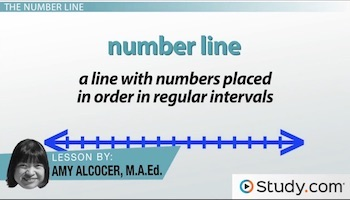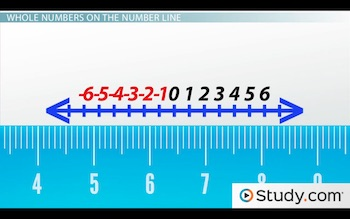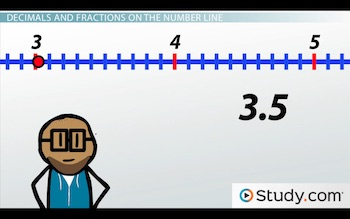# Using the Number Line to Compare Decimals, Fractions, and Whole Numbers

An error occurred trying to load this video.

Try refreshing the page, or contact customer support.

Coming up next: How to Solve Complex Fractions

### You're on a roll. Keep up the good work!

Replay
Your next lesson will play in 10 seconds
• 0:01 The Number Line
• 0:32 Whole Numbers on the…
• 1:40 Decimals and Fractions…
• 4:47 Comparing the Numbers…
• 5:31 Lesson Summary
Save Save

Want to watch this again later?

Timeline
Autoplay
Autoplay
Speed Speed

#### Recommended Lessons and Courses for You

Lesson Transcript
Instructor: Yuanxin (Amy) Yang Alcocer

Amy has a master's degree in secondary education and has taught math at a public charter high school.

Watch this video lesson to learn how useful the number line can be when you need to compare two different numbers, be they whole numbers, decimals or even fractions. Learn how to plot these numbers on the number line so you can compare them.

## The Number Line

We begin with our number line, a line with numbers placed in order in regular intervals.Think of it as a ruler. It shows you the proper order of numbers. Knowing how to use a number line is one of the most basic math skills, and it gives you a good foundation upon which to grow your math skills. It is in this video lesson that you will learn how to find numbers on the number line, to plot numbers on the line, and then to compare these numbers. So, are you ready to get going? Then let's go!

## Whole Numbers on the Number Line

We first tackle whole numbers on the number line. Recall that whole numbers are your counting numbers, like 1, 2, 3 and so on.

Finding whole numbers on the number line is fairly easy. Why is this? Well, if you look at your number line or even a ruler, what do you see?Why, you see your whole numbers. You see 0 in the middle of the number line. To the right, you see your positive numbers. Going to the right from 0, we have 1, 2, 3, 4 and so on. You keep going to the right and your numbers keep getting bigger.

Going to the left from 0, we have our negative numbers, and these keep getting more and more negative. We have -1, -2, -3 and so on. So, if we wanted to find the number 5, for instance, what do you think we would have to do? Yes, we would go the right 5 spaces from 0 to find our 5.

To plot whole numbers, we use a similar procedure. If we wanted to plot 3, we would start at 0 and then go three spaces to the right to find where our 3 is located. At this point, we place our dot.

## Decimals and Fractions on the Number Line

Now, what about decimals (numbers with a decimal point) and fractions (numbers representing a part of a whole)? How do we find and plot these numbers? For these types of numbers, first we have to realize that they tell you that your number is more than a whole number. These numbers have a part of a whole number included. For example, the decimal 3.5 tells you that you have three whole things and then half a thing. If we thought about it in terms of pies, we would have three whole pies and then half of a pie. So, the numbers after the decimal point tell you how much of another whole you have - how much of another pie you have.

Fractions are related to decimals, as you can go back and forth between fractions and most decimals. For example, the fraction 3 1/2 can be converted to our decimal 3.5. We made the conversion by dividing the 1 and the 2 and then adding it to the 3. This is the kind of conversion we would make for mixed fractions. If we had the fraction 7/2, on the other hand, we would do a straight division to find our decimal. 7 divided by 2 is also 3.5. All these numbers are the same.

The way that makes the most sense for me when looking for fractions on a number line is to convert these to decimals first and then to look for them. And this is what I want you to do. Convert your fractions to decimal before working with them on the number line.

To find your decimal or fraction turned into a decimal on the number line, we first find our whole number part and then we figure out how much more we need to go for the part after the decimal, the part of a whole. For example, to find the number 3.5, we first find our 3. We find this by going 3 spaces to the right of 0. Now we need to figure out how much we have to go to account for the .5 after the three. To do this, we can put little slash marks between our 3 and 4 to divide this section into 10 spaces.To unlock this lesson you must be a Study.com Member.

### Register to view this lesson

Are you a student or a teacher?

#### See for yourself why 30 million people use Study.com

##### Become a Study.com member and start learning now.
Back
What teachers are saying about Study.com

### Earning College Credit

Did you know… We have over 200 college courses that prepare you to earn credit by exam that is accepted by over 1,500 colleges and universities. You can test out of the first two years of college and save thousands off your degree. Anyone can earn credit-by-exam regardless of age or education level.